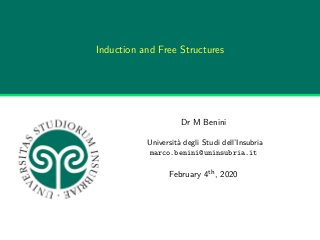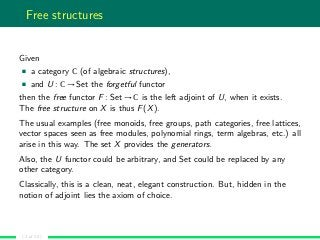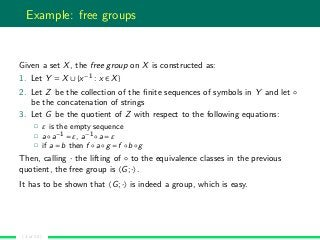Successfully reported this slideshow.

# Induction and Free Structures

0

Share×
1 of 20
1 of 20

# Induction and Free Structures

0

Share

Free structures pervade Mathematics: free monoids, free groups, the natural numbers are just the most obvious examples. These structures are characterised by a set of generators coupled with a generating process which allows to combine the generators with no constraints, roughly speaking. The generation process naturally yields an induction principle for the free structure: if a property holds for all the generators and it preserves the generating process, then the property holds for every element in the free structure. In this respect, induction can be thought of as an algebraic action of the free structure over some domain.
The talk aims at illustrating and exploring induction as an algebraic action, drawing a few, very preliminary consequences mainly through examples.

Free structures pervade Mathematics: free monoids, free groups, the natural numbers are just the most obvious examples. These structures are characterised by a set of generators coupled with a generating process which allows to combine the generators with no constraints, roughly speaking. The generation process naturally yields an induction principle for the free structure: if a property holds for all the generators and it preserves the generating process, then the property holds for every element in the free structure. In this respect, induction can be thought of as an algebraic action of the free structure over some domain.
The talk aims at illustrating and exploring induction as an algebraic action, drawing a few, very preliminary consequences mainly through examples.

## More Related Content

### Related Books

Free with a 14 day trial from Scribd

See all

### Related Audiobooks

Free with a 14 day trial from Scribd

See all

### Induction and Free Structures

1. 1. Induction and Free Structures Dr M Benini Università degli Studi dell’Insubria marco.benini@uninsubria.it February 4th, 2020
2. 2. Free structures Given a category C (of algebraic structures), and U : C → Set the forgetful functor then the free functor F : Set → C is the left adjoint of U, when it exists. The free structure on X is thus F(X). The usual examples (free monoids, free groups, path categories, free lattices, vector spaces seen as free modules, polynomial rings, term algebras, etc.) all arise in this way. The set X provides the generators. Also, the U functor could be arbitrary, and Set could be replaced by any other category. Classically, this is a clean, neat, elegant construction. But, hidden in the notion of adjoint lies the axiom of choice. ( 2 of 20 )
3. 3. Example: free groups Given a set X, the free group on X is constructed as: 1. Let Y = X ∪{x−1 : x ∈ X} 2. Let Z be the collection of the ﬁnite sequences of symbols in Y and let ◦ be the concatenation of strings 3. Let G be the quotient of Z with respect to the following equations: ε is the empty sequence a◦a−1 = ε, a−1 ◦a = ε if a = b then f ◦a◦g = f ◦b ◦g Then, calling · the lifting of ◦ to the equivalence classes in the previous quotient, the free group is 〈G;·〉. It has to be shown that 〈G;·〉 is indeed a group, which is easy. ( 3 of 20 )
4. 4. Example: free Heyting algebras Given a set X, the free Heyting algebra on X is constructed as: 1. Let Z be the set of formulae in the intuitionistic propositional language over the variables X 2. Let ∼ be the relation on Z given by a ∼ b if and only if a b and b a 3. Let H be the quotient of Z with respect to ∼ Then, calling ≤ the relation on H deﬁned by [a] ≤ [b] if and only if a b, the free Heyting algebra is 〈H;≤〉. It has to be shown that 〈H;≤〉 is indeed a Heyting algebra, which is the core of the construction behind the completeness theorem for intuitionistic propositional logic. ( 4 of 20 )
5. 5. Example: natural and integer numbers Z is the free additive group generated by 1. N is the free additive monoid generated by the 1. These deﬁnitions use in an essential way the unary representation of numbers. ( 5 of 20 )
6. 6. Example: the torus The torus seen as an homotopy space is deﬁned as b : T, the torus contains a base point l1,l2 : b =T b, there are two loops from b to b, and they are not the identity path h : l1 ◦l2 =b=Tb l2 ◦l1, there is a non-trivial homotopy from l1 ◦l2 to l2 ◦l1, with ◦ path composition This example uses homotopy type theory and, in particular, the interpretation of witnesses in α =A α as paths from α to α. ( 6 of 20 )
7. 7. Constructions In each of the previous examples, the free object is constructed from a collection Z of syntactical objects1 in which Z is reﬁned to obtain the free object23 The construction follows a somewhat general pattern. In this respect, we can regard homotopy type theory as an attempt to regularise this construction keeping track of the proof-relevant pieces of information. 1In the case of the torus, Z is formed by constructing the judgements about T and its equality types 2For groups, Heyting algebras, and numbers, the reﬁnement is a quotient 3For the torus, the reﬁnement passes through identiﬁcation of paths and homotopies in type theory ( 7 of 20 )
8. 8. Remarks It is worth noting that the construction envisages a sort of completeness result, see Heyting algebras. It suggests that the free object is a classifying model for all the others objects of the same sort: if a property holds in the free model then it holds in every model because every other objects can be constructed from the free one by truth-preserving maps. the construction envisages a sort of normalisation result. It suggests that the elements in the free object are either values or expressions, and the latter reduce to the former via the reﬁnement from Z (see the previous slide) to the free object. ( 8 of 20 )
9. 9. Completeness The remark about completeness is obvious for Heyting algebras, leading to the completeness theorem for intuitionistic propositional logic. In group theory, the same remark means that every group is the quotient of a free group. Also, the proof of Cayley’s theorem, every group is a subgroup of a symmetric group, can be stated as a consequence of the “completeness” of groups: the usual proof based on actions is performed on the free group (making it concrete), and we observe that a subgroup of a subgroup of a group is a subgroup of the group, also showing that the symmetric group of a group is the quotient of the symmetric group of the free group via the same quotient. ( 9 of 20 )
10. 10. Normalisation The fundamental group of the 1-sphere is the free group generated by a non-trivial loop on some base point. Consider its construction: every composition of loops from the point is an “expression” and the group forces it to reduce to an irreducible “value”. Proving that π1(S1) is the additive group Z is as simple as noting that Z ∼ { n : n ∈ Z} and the second set is the collection of “values” which turns out to be a group with path concatenation as its operation. Proving that π1(T) ∼ Z×Z, the fundamental group of the torus is Z×Z with pointwise addition follows the same pattern. This are, in fact, normalisation theorems. ( 10 of 20 )
11. 11. Induction An induction principle codes the previous construction in a (set of) inference rule(s). In fact, it does more: it codes the lifting of the previous construction in the space of propositions. More in general, induction lifts the construction in some space: propositions, functions, . . . We may think that the free structure acts on the space, in analogy with the action of a group on a set. ( 11 of 20 )
12. 12. Induction Two important remarks are due diﬀerent representations of the same free object, although equivalent, may be very distant diﬀerent ways of constructing the free object lead to diﬀerent induction principles, which are not necessarily equivalent As a consequence, the action of induction over the space of propositions, functions, etc., may be radically diﬀerent, depending on the way in which the free object construction is coded. ( 12 of 20 )
13. 13. Example: simple theory of types Consider Church’s simple theory of types. The strong normalisation theorem states: For every term t : T, every reduction starting from t admits only ﬁnite extensions. The obvious attempt is to try to prove the statement by induction on the derivation of t : T. This attempt (and many variations one could think of) fail. The problem lies in β-reduction: if λx : A.b : A → B and a : A are derivable, then (λx : A.b)a β b[a/x] and we have to show that b[a/x] : B is strongly normalisable. It is easy to show that b[a/x] : B is derivable, and it is easy to show that b : B whenever x : A, but b[a/x] may be constructed after b, a, and λx : A.b, preventing to use the induction hypothesis. ( 13 of 20 )
14. 14. Example: simple theory of types Tait and Girard proposed a proof which circumvents the problem using a stronger form of induction. Their principle scans the free algebra of terms in a diﬀerent way: for an atomic type T, its terms are collected; for a product type A×B, its terms are the pairs (π1 t,π2 t) with t : A×B; for a function space A → B, its terms are the abstractions λx : A.t x with x new and t a : B for every a : A. The corresponding action allows to prove the strong normalisation theorem in a more or less straightforward way. The fundamental observation is that the same free object can be presented in a diﬀerent but more convenient way, essentially following the inductive structure of types (and using the η-equivalences). ( 14 of 20 )
15. 15. A modest application Syntactically, homotopy type theory can be described as the intensional version of Martin-Löf’s type theory with a hierarchy of universes, plus two axioms, univalence and function extensionality, plus higher inductive types. It is folklore that this version of Martin-Löf’s type theory enjoys the strong normalisation property. In fact, a real proof lacks. In her PhD thesis, R. Bonacina proved (under my supervision) a strong normalisation theorem for a large fragment of homotopy type theory, which comprehends Martin-Löf’s type theory, univalence, function extensionality, and a large family of higher inductive types. The result states that considering judgemental equality as a convertibility relation, every reduction from a judgement cannot be indeﬁnitely extended. ( 15 of 20 )
16. 16. A modest application The formal system is huge and open (the collection of types is not ﬁxed, although they share a common pattern). Also, the presence of dependent types and multiple universes make the usual approaches, e.g., those working for the simple theory of types, not immediate to extend. Therefore, our proof is long and technically complex, beyond my ability to present it in a conference talk. However, it is based on Tait’s technique, and it is an obvious extension of the proof about the simple theory of types, wearing the right set of glasses. ( 16 of 20 )
17. 17. A modest application The ﬁrst part is to construct the set Z of all derivable judgements, by combining in all the possible ways the inference rules. As before, the set Z forms the basis on which we construct a free object. Then, we quotient this object using judgemental equality: this is the free object we are interested in. We want to show that in each equivalence class there is at least one irreducible element, and every reduction from any element in the class reaches it in a ﬁnite number of steps. The construction of the free object comes with an induction principle: the usual induction on the structure of formal proofs. Our ﬁrst attempts to prove the strong normalisation theorem used this induction principle. We knew in advance that these attempts were bound to failure. But the way in which they failed was what we were seeking. ( 17 of 20 )
18. 18. A modest application The failures showed that, in essence, the bad behaviour of the induction principle was the same as in the simple theory of types, except that the whole framework was far more complex. Then, we started to play with Girard’s variant of the notion of reducibility candidates. Apart its formal deﬁnition, the notion deﬁnes the desired action the induction principle should perform on judgements in order to prove the normalisation theorem. The idea is to scan the free object so that the structure of types governs the order in which the terms are examined. As in the case of the simple theory of types, the eﬀect is that we can apply the induction hypotheses exactly when we need them. ( 18 of 20 )
19. 19. A modest application Precisely, if a type converts to a variable, its terms are the terms of its possible instances. if a type does not convert to a variable, but it converts to a dependent product Πx : A.B, its terms are the abstractions λx : A.t x with x new and t a : B[a/x] for every a : A. otherwise, the type is atomic, and its terms are collected. The corresponding induction principle allows to prove the normalisation theorem following Girard’s approach. The proof is long and tedious but not too diﬃcult. The induction principle works because the above scan is well founded, which is a consequence that universes are atomic types (proved via semantics). Uniqueness of irreducible judgements follows assuming Π to be injective, which is proved via semantics. ( 19 of 20 )
20. 20. The end Questions? ( 20 of 20 )